# Math in Focus Grade 6 Chapter 6 Lesson 6.1 Answer Key Understanding Percent

Practice the problems of Math in Focus Grade 6 Workbook Answer Key Chapter 6 Lesson 6.1 Understanding Percent to score better marks in the exam.

## Math in Focus Grade 6 Course 1 A Chapter 6 Lesson 6.1 Answer Key Understanding Percent

### Math in Focus Grade 6 Chapter 6 Lesson 6.1 Guided Practice Answer Key

Solve.

Question 1.
Out of 25 chairs, 14 are brown. What percent of the chairs are brown?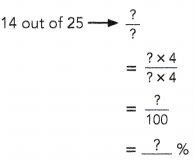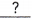% of the chairs are brown.
56% of the chairs are brown.
Explanation:
Out of 25 chairs, 14 are brown.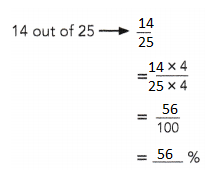Question 2.
Of the 400 animals in a zoo, 32 were monkeys.
a) What percent of the animals were monkeys?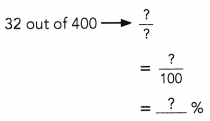% of the animals were monkeys.
8% of the animals were monkeys.
Explanation: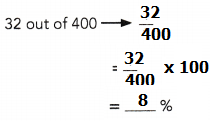b) What percent of the animals were not monkeys?% –% =%% of the animals were not monkeys.
92 % of the animals were not monkeys.
Explanation:
8% of the animals were monkeys.
100 % – 8% = 92 %

Express each percent as a fraction or a mixed number in simplest form.

Question 3.
48% = $$\frac{48}{?}$$
= $$\frac{?}{?}$$
$$\frac{12}{25}$$
Explanation:
48% = $$\frac{48}{100}$$
= $$\frac{12 . 4}{25 . 4}$$
= $$\frac{12}{25}$$

Question 4.
55%
$$\frac{11}{20}$$
Explanation:
55% = $$\frac{55}{100}$$
= $$\frac{11 . 5}{20 . 5}$$
= $$\frac{11}{20}$$

Question 5.
108%
$$\frac{108}{100}$$
Explanation:
108% = $$\frac{108}{100}$$
= $$\frac{52 . 4}{25 . 4}$$
= 2$$\frac{2}{25}$$

Express each percent as a decimal.

Question 6.
13% = $$\frac{?}{?}$$
=0.13
Explanation:
13% = $$\frac{13}{100}$$
= 0.13

Question 7.
8%
0.08
Explanation:
8% = $$\frac{8}{100}$$
= 0.08

Question 8.
126%
1.26
Explanation:
126% = $$\frac{126}{100}$$
=1.26

### Math in Focus Course 1A Practice 6.1 Answer Key

Question 1.
Out of a total of 500 flowers, 65 are roses. What percent of the flowers are roses?
13%
Explanation: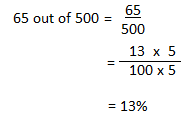Question 2.
Of the 200 packages of bagels sold, 15 of them are sesame seed bagels. What percent of the bagel packages sold are sesame seed bagels?
7.5%
Explanation:
15 out of 200 = $$\frac{15}{200}$$
= $$\frac{15}{2 X 100}$$
= $$\frac{7.5}{100}$$

Question 3.
In a survey of music preferences, 81 out of 450 students said that they preferred country music. What percent of the students preferred country music?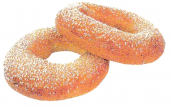18%
Explanation: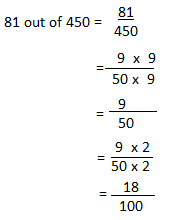Question 4.
There are 750 spectators in the stadium, of which 420 are women and the rest are men.
a) What percent of the spectators are women?
56%
Explanation:
420 out of 750 =
= $$\frac{420}{750}$$
= $$\frac{42}{75}$$
= 0.56 x 100 = 56%

b) What percent of the spectators are men?
44%
Explanation:
420 out of 750 =
= $$\frac{420}{750}$$
= $$\frac{42}{75}$$
= 0.56 x 100
percent of women spectators = 56%
percent of men spectators = 100 – 57 = 44%

Express each percent as a fraction or a mixed number in simplest form.

Question 5.
65%
$$\frac{13}{20}$$
Explanation: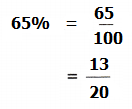Question 6.
78%
$$\frac{39}{50}$$
Explanation:
78% = $$\frac{78}{100}$$
Divided numerator and denominator with 2
= $$\frac{39}{50}$$

Question 7.
92%
$$\frac{23}{25}$$
Explanation:
92% = $$\frac{92}{100}$$
Divided both the numerator and denominator with 4
= $$\frac{23}{25}$$

Question 8.
125%
$$\frac{5}{4}$$
Explanation:
125% = $$\frac{125}{100}$$
Divided numerator and denominator with 5
= $$\frac{5}{4}$$

Question 9.
276%
$$\frac{66}{25}$$
Explanation:
276% = $$\frac{276}{100}$$
Divided numerator and denominator with 4
= $$\frac{66}{25}$$

Question 10.
580%
$$\frac{29}{5}$$
Explanation:
580% = $$\frac{580}{100}$$
Divided numerator and denominator with 2
= $$\frac{29}{5}$$

Express each percent as a decimal.

Question 11.
6%
0.06
Explanation:
In order to convert percent to decimal number,
percentage should be divided by 100.
$$\frac{6}{100}$$ = 0.06

Question 12.
43%
0.43
Explanation:
In order to convert percent to decimal number,
percentage should be divided by 100.
$$\frac{43}{100}$$ = 0.43

Question 13.
80%
0.8
Explanation:
In order to convert percent to decimal number,
percentage should be divided by 100.
$$\frac{80}{100}$$ = 0.8

Question 14.
367%
3.67
Explanation:
In order to convert percent to decimal number,
percentage should be divided by 100.
$$\frac{367}{100}$$ = 3.67

Question 15.
579%
5.79
Explanation:
In order to convert percent to decimal number,
percentage should be divided by 100.
$$\frac{579}{100}$$ = 5.79

Question 16.
779%
7.79
Explanation:
In order to convert percent to decimal number,
percentage should be divided by 100.
$$\frac{779}{100}$$ = 7.79

Question 17.
The music for Nadia’s dance routine lasts for exactly 4 minutes. When Nadia dances her routine, she starts with her music and finishes 12 seconds before the music ends. What percent of the time the music is playing is Nadia dancing?
95%
Explanation:
4 minutes is 4 x 60 seconds = 240 seconds.
Nadia stops 12 seconds before the music ends so 240 – 12 = 228 seconds she dances.
= $$\frac{228}{240}$$ = 0.95
0.95 x 100 = 95%

Question 18.
Math Journal In a game of darts, Annie hits the bull’s eye 4 times out of 25 times. Benjamin hits the bull’s eye 8 times out of 100 times.
a) Who hits the bull’s eye more times?
$$\frac{8}{100}$$ = 8%
= $$\frac{4}{25}$$
= $$\frac{4 X 4}{25 X 4}$$
$$\frac{16}{100}$$ = 16%